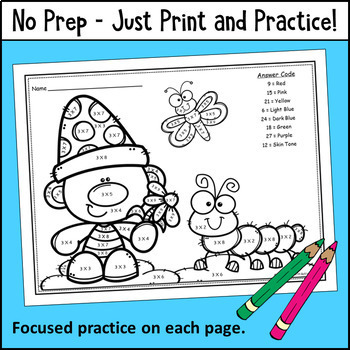# Multiplication Fact Practice Color by Number Free Printable Math Worksheets

Rated 4.74 out of 5, based on 323 reviews
323 Ratings;
3rd - 4th
Subjects
Resource Type
Standards
Formats Included
• PDF
Pages
Report this resource to TPT

### Description

A collection of 11 Multiplication Color by Number pages with a fun spring theme! The set includes a focus page for each of the multiples of 2 through 12. Works well to print and send home in packets for distance learning and at-home learning. Answer keys are included.

The pages in this set are differentiated by factors to make it easy to select the appropriate pages for your students. You may use all of the pages with your entire class or differentiate based on ability-level. Your students will have fun practicing their multiplication facts with these pages!

Color by Number Pages:

Multiply by 2

Multiply by 3

Multiply by 4

Multiply by 5

Multiply by 6

Multiply by 7

Multiply by 8

Multiply by 9

Multiply by 10

Multiply by 11

Multiply by 12

Looking for a more advanced multiplication color by number? Use the following link to view my multi-digit multiplication version:

Multi-Digit Multiplication Color by Number – Spring Edition

Other resources you may like:

Multiples Coloring Pages

Monster Products Third Grade Multiplication Card Game

Monster Products & Quotients Card Game BUNDLE

FREE My Book of Tenths

To catch freebies and new resources:

Follow the Mitchell MATH Activities Blog

Follow Mitchell MATH Activities on TPT

Follow Mitchell MATH Activities on Pinterest

I am interested in hearing your feedback!

Thank you,

Janet Mitchell

Total Pages
Included
Teaching Duration
N/A
Report this resource to TPT
Reported resources will be reviewed by our team. Report this resource to let us know if this resource violates TPT’s content guidelines.

### Standards

to see state-specific standards (only available in the US).
Determine the unknown whole number in a multiplication or division equation relating three whole numbers. For example, determine the unknown number that makes the equation true in each of the equations 8 × ? = 48, 5 = __ ÷ 3, 6 × 6 = ?.
Fluently multiply and divide within 100, using strategies such as the relationship between multiplication and division (e.g., knowing that 8 × 5 = 40, one knows 40 ÷ 5 = 8) or properties of operations. By the end of Grade 3, know from memory all products of two one-digit numbers.